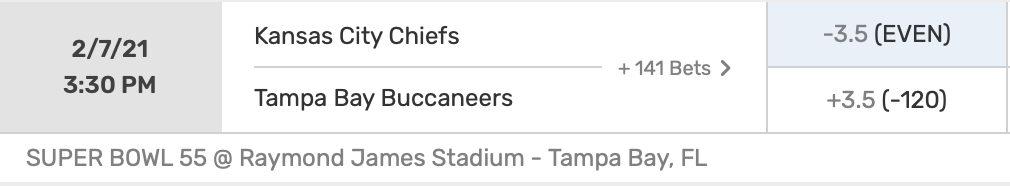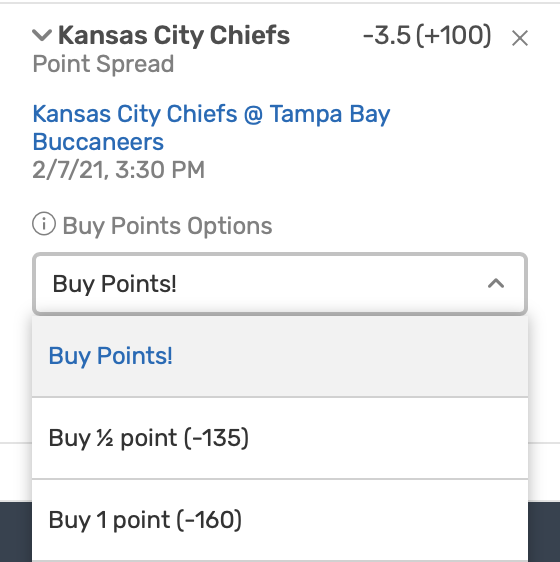# Why You Shouldn’t Buy Points for Super Bowl 2021.

### Understanding the Question.

In 2021, we have been blessed with the Super Bowl matchup of our dreams, G.O.A.T. Brady vs. God Mahomes. The opening line on Bovada is Chiefs -3.5 (+100) and Bucs +3.5 (-120), as shown in the screenshot below.I’ll be betting on the Chiefs because clearly they’re the better team, and I’ll take a 25 year-old Mahomes over a 43 year-old Brady. However, the -3.5 line gives me pause, because 3 points is the most common margin of victory in the NFL, and I don’t want to lose my bet via the Chiefs’ winning the Super Bowl by 3. So, Bovada provides some alternatives: I can “buy points”. In other words, Bovada has alternative lines, including the Chiefs -3 (-135) and the Chiefs -2.5 (-160), as indicated by the screenshot below.So, is it worth it to pay the stiff juice of a $$-135$$ or $$-160$$ moneyline to avoid despair if the Chiefs win by a field goal?

### Probability Model.

Let $$X_0$$ be the random variable denoting the profit from betting $$\B$$ on the original line, Chiefs -3.5 (+100):

$X_0 = \begin{cases} +B & \text{if Chiefs win by } \geq 4 \\ -B & \text{else}. \\ \end{cases}$

Let $$X_{1/2}$$ denote the profit from betting $$\B$$ on the “buy $$1/2$$ point” line, Chiefs -3 (-135):

$X_{1/2} = \begin{cases} +\frac{100}{135}B & \text{if Chiefs win by } \geq 4 \\ 0 & \text{if Chiefs win by exactly } 3 \\ -B & \text{else}. \\ \end{cases}$

Let $$X_1$$ denote the profit from betting $$\B$$ on the “buy $$1$$ point” line, Chiefs -2.5 (-160):

$X_1 = \begin{cases} +\frac{100}{160}B & \text{if Chiefs win by } \geq 3 \\ -B & \text{else}. \\ \end{cases}$

To make the model more explicit, we introduce 2 parameters. Let $$p$$ denote the probability that the Chiefs win by $$\geq 4$$ points, and let $$q$$ denote the probability that the Chiefs win by exactly $$3$$ points. Then

$X_0 = \begin{cases} +B & \text{with probability } p \\ -B & \text{with probability } 1-p \end{cases}$

$X_{1/2} = \begin{cases} +\frac{100}{135}B & \text{with probability } p \\ 0 & \text{with probability } q \\ -B & \text{with probability } 1-p-q \\ \end{cases}$

$X_1 = \begin{cases} +\frac{100}{160}B & \text{with probability } p+q \\ -B & \text{with probability } 1-p-q. \\ \end{cases}$

The most profitable strategy has the highest expected profit. So, we will compare $$\mathbb{E}X_0,\mathbb{E}X_{1/2},\text{ and }\mathbb{E}X_1$$, and choose the strategy with the highest such value. Well,

\begin{aligned} \mathbb{E}X_0 &= B(p) - B(1-p) \\ &= B(2p-1)\\ \\ \mathbb{E}X_{1/2} &= B(\frac{100}{135}p) - B(1-p-q) \\ &= B(\frac{47}{27}p + q - 1) \\ \\ \mathbb{E}X_1 &= B(\frac{100}{160}(p+q)) - B(1-p-q) \\ &= B(\frac{13}{8}p+\frac{13}{8}q-1). \end{aligned}

Now, we need only calculate $$p$$ and $$q$$.

### Estimating $$p$$ and $$q$$.

Given that the Chiefs are a $$3.5$$ point favorite, $$p$$ is the probability that the Chiefs win by $$\geq 4$$ points, and $$q$$ is the probability that the Chiefs win by exactly $$3$$ points. Our task is to estimate $$p$$ and $$q$$.

I found a dataset containing the opening and closing pointspreads from every NFL game during the last 10 years, ending with NFL Week 14, 2020. Here is a peek at the head of the dataset, showing the some of the Week 14 results.

        date away.team home.team away.score home.score home.line.open home.line.close
1 2020-12-14    Ravens    Browns         47         42            1.0             3.0
2 2020-12-13  Steelers     Bills         15         26            2.0            -2.0
3 2020-12-13   Falcons  Chargers         17         20            2.5            -1.0
4 2020-12-13    Saints    Eagles         21         24            7.0             7.5

Now, we narrow the dataset to include only the games that had an opening or closing pointspread of $$3.5$$. Then, we modify the dataset to include 2 new columns: fave.covers, which is TRUE iff the favorite covered the $$-3.5$$ spread, and exactly.3, which is TRUE iff the final score ended in the favorite winning by exactly 3 points. Here is a peek at the head of the updated dataset, omiting the column home.line.close to save space for aesthetics.

          date away.team  home.team away.score home.score home.line.open fave.covers exactly.3
1   2020-12-13   Broncos   Panthers         32         27           -3.5       FALSE     FALSE
286 2020-12-06     Colts     Texans         26         20            2.5        TRUE     FALSE
2   2020-12-06    Browns     Titans         41         35           -4.0       FALSE     FALSE
3   2020-12-02    Ravens   Steelers         14         19           -3.5        TRUE     FALSE
287 2020-11-29    Chiefs Buccaneers         27         24            3.5       FALSE      TRUE
4   2020-11-23      Rams Buccaneers         27         24           -3.5       FALSE     FALSE

Interestingly, as shown above, the Chiefs and Bucs met earlier in the season, on November 29, 2020. The Chiefs were also $$3.5$$ point favorites then, and they won by exactly 3 points! Spooky!

Now, we estimate $$p$$, the probability that a $$-3.5$$ point favorite covers the spread, and $$q$$, the probability that a $$-3.5$$ point favorite wins by exactly 3 points, by the empirical proportions from our dataset. Hence

$p = 0.48$

and

$q = 0.08.$

Finally, note that our dataset of games having a pointspread of $$3.5$$ contains $$463$$ games, which is a nice sample size, enough to give us some confidence in our estimates for $$p$$ and $$q$$.

### Conclusion.

As calculated previously,

$\begin{cases} \mathbb{E}X_0 = B(2p-1)\\ \mathbb{E}X_{1/2} = B(\frac{47}{27}p + q - 1) \\ \mathbb{E}X_1 = B(\frac{13}{8}p+\frac{13}{8}q-1). \end{cases}$

Using $$p = 0.48$$ and $$q = 0.08$$, we get

$\begin{cases} \mathbb{E}X_0 = -0.04B \\ \mathbb{E}X_{1/2} = -0.08B \\ \mathbb{E}X_1 = -0.09B. \end{cases}$

To make this calculation more concrete, we choose a value for $$B$$. Since Ryan Brill and his buddy Nick Miller made $$\ 50$$ worth of NFL bets each week on the 2020 edition of the Bet, Sweat, and Forget Podcast, we shall use $$B =50$$, yielding

$\begin{cases} \mathbb{E}X_0 = -2 \\ \mathbb{E}X_{1/2} = -4.22 \\ \mathbb{E}X_1 = -4.5. \end{cases}$

So, betting the original line is the most profitable strategy, because its expected profit is the least negative. In fact, betting $$\50$$ and not buying points saves you at least $$\ 2.22$$ on average.

### More General Moneylines.

More generally, we suppose that the original pointspread has a moneyline of $$-M_0$$ for the favorite, the “buy a half point” spread has a moneyline of $$-M_{1/2}$$, and the “buy a point” spread has a moneyline of $$-M_1$$. Suppose we bet $$\ B$$. Let $$p$$ be the probability that the favorite covers in the original bet, and let $$q$$ be the probability that the final game score is exactly the pointspread in the “buy a half point” bet. Then the profits $$X_0, X_{1/2}, \text{ and } X_1$$ of betting on the respective pointspreads are

$X_0 = \begin{cases} +B\frac{100}{M_0} & \text{with probability } p \\ -B & \text{with probability } 1-p \end{cases}$

$X_{1/2} = \begin{cases} +\frac{100}{M_{1/2}}B & \text{with probability } p \\ 0 & \text{with probability } q \\ -B & \text{with probability } 1-p-q \\ \end{cases}$

$X_1 = \begin{cases} +\frac{100}{M_1}B & \text{with probability } p+q \\ -B & \text{with probability } 1-p-q. \\ \end{cases}$

The expected profits are thus

$\begin{cases} \mathbb{E}X_0 = B\frac{100}{M_0} p - B (1-p) \\ \mathbb{E}X_{1/2} = B\frac{100}{M_{1/2}} p -B (1-p-q)\\ \mathbb{E}X_1 = B\frac{100}{M_1} (p+q) -B (1-p-q). \\ \end{cases}$

The most profitable strategy has the highest expected profit.